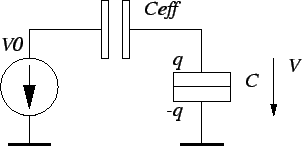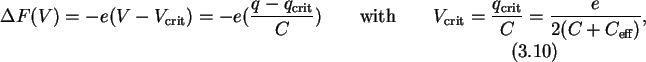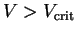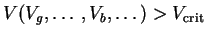Next: 3.2 Tunneling: a Stochastic Up: 3.1 Free Energy of Previous: 3.1.4 Free Energy

## 3.1.5 Method of Critical Voltage

The first term for the calculation of the change in free energy in (3.8) is a quadratic form, a vector-matrix-vector multiplication which needs 2N2-1 scalar operations (multiplications and additions) for its evaluation. It is possible to reduce this expenditure slightly to 2N2-N-1 operations by employing the method of critical voltage or   critical charge    . Since this is only a linear reduction for a quadratic expression it will not matter much for large circuits. However the concept of a critical charge, as is shown in the following, may help in understanding the operation of more complex circuits.

Asking what free energy change a tunnel event in a particular junction causes, one may look at the equivalent circuit Fig. 3.4 using Thévenin's theorem.The change in free energy, which includes electrostatic energy and work done by the voltage source, may be written as a linear function of the voltage across the tunnel junction V, or, what is equivalent, as a function of the charge q on the tunnel junction.whereis the   effective capacitance of the  Thévenin equivalent circuit. The critical voltage is computed once for every junction outside the inner simulation loop. In the inner loop only node potentials are calculated (3.2), which is a matrix-vector multiplication, from which the voltage across tunnel junctions and finally, using the critical voltage, the change in free energy (3.10) is calculated. Studying the changing voltage across a tunnel junction in comparison to the constant critical voltage may lead to a better understanding when tunnel rates are likely and when not. It is possible to use the critical voltage as the basis to the determination of  stable and  instable regions of device operation . For zero temperature (3.10) gives the region where tunneling is allowed as. V usually is a function of some external gate and bias voltage sources. Thendefines a border between a stable and an instable region. In general the border is an n-dimensional plane, with n being the number of independent quantities V is depending on. Every tunnel junction defines such a plane. Altogether they mark regions of stable and instable operation.Next: 3.2 Tunneling: a Stochastic Up: 3.1 Free Energy of Previous: 3.1.4 Free Energy

Christoph Wasshuber